# To graph : The quadratic equation y = x 2 + 7 x + 6 in a graphing window.### Precalculus: Mathematics for Calcu...

6th Edition
Stewart + 5 others
Publisher: Cengage Learning
ISBN: 9780840068071### Precalculus: Mathematics for Calcu...

6th Edition
Stewart + 5 others
Publisher: Cengage Learning
ISBN: 9780840068071

#### Solutions

Chapter 1.9, Problem 6E
To determine

## To graph: The quadratic equation y=x2+7x+6 in a graphing window.

Expert Solution

### Explanation of Solution

Given information:

Graph:

The graph of the quadratic equation y=x2+7x+6 can be sketched in the cartesian plane,

Consider the quadratic equation, y=x2+7x+6 .

Now put the values of x in the equation to find the values of y or to find the real roots of y with the help of x .

The method of middle term factorisation is used in the equation y=x2+7x+6 :

y=x2+7x+6y=x2+6x+x+6y=x(x+6)+1(x+6)y=(x+1)(x+6)

Now either x+1=0 or x+6=0 .

So x=1 or x=6 ,

Here observed the equation of parabola, the coefficient of x2 is positive, so the graph is opened upward.

The roots and the shape of parabola is cleared.

The graph of the function y=x2+7x+6 .

Substitute x=0 , in the equation y=x2+7x+6 ,

y=x2+7x+6y=(0)2+7(0)+6y=6

Substitute x=1 , in the equation y=x2+7x+6 ,

y=x2+7x+6y=(1)2+7(1)y=1+7y=8

Substitute x=2 , in the equation y=x2+7x+6 ,

y=x2+7x+6y=(2)2+7(2)+6y=4+14+6y=24

Observe that as the value of x increases there is the increment in value of y .

Steps to plot the graph of the equation y=x2+7x+6 with the help of graphing utility are as follows:

Step 1: Press MODE key.

Step 2: Use the down arrow key to reach FUNC option.

Step 3: Press ENTER key.

Step 4: Press Y= key.

Step 5: Enter the function y=x2+7x+6 .

Step 6: Press WINDOW key. Change the settings to

Xmin=10Xmax=10Ymin=10Ymax=10

For better view of graph.

Step 8: Press GRAPH key.

The result obtained on the screen is provided below,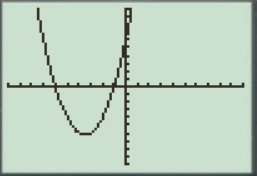Interpretation:

The equation of the function y=x2+7x+6 represents a parabola.

The parabola opens upward.

The x intercepts are the points on xaxis where the graph of the equation touches xaxis .

Therefore, in the equation, y=x2+7x+6 there is no x intercepts.

Therefore, the equation y=x2+7x+6 is symmetric about the y axis Initially the graph of the function y=x2+7x+6 increases when increase the value of x ,the graph is a parabola shaped graph.

a.

When the x -axis ranges from [5,5] and y -axis ranges from [5,5] their graph obtained in the screen is provided below.

Steps to plot the graph of the equation y=x2+7x+6 with the help of graphing utility are as follows:

Step 1: Press MODE key.

Step 2: Use the down arrow key to reach FUNC option.

Step 3: Press ENTER key.

Step 4: Press Y= key.

Step 5: Enter the function y=x2+7x+6 .

Step 6: Press WINDOW key. Change the settings to

Xmin=5Xmax=5Ymin=5Ymax=5

For better view of graph.

Step 8: Press GRAPH key.

The result obtained on the screen is provided below,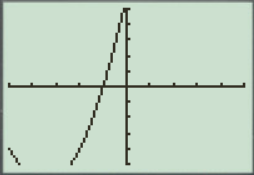This window is not perfect viewing window.

b.

When the x -axis ranges from [0,10] and y -axis ranges from [20,100] their graph obtained in the screen is provided below.

Steps to plot the graph of the equation y=x2+7x+6 with the help of graphing utility are as follows:

Step 1: Press MODE key.

Step 2: Use the down arrow key to reach FUNC option.

Step 3: Press ENTER key.

Step 4: Press Y= key.

Step 5: Enter the function y=x2+7x+6 .

Step 6: Press WINDOW key. Change the settings to

Xmin=0Xmax=10Ymin=20Ymax=100

For better view of graph.

Step 8: Press GRAPH key.

The result obtained on the screen is provided below,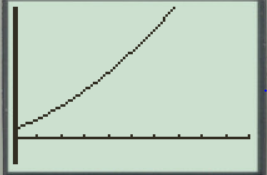This window is not a perfect viewing window.

c.

When the x -axis ranges from [15,8] and y -axis ranges from [20,100] their graph obtained in the screen is provided below.

Steps to plot the graph of the equation with the help of graphing utility are as follows:

Step 1: Press MODE key.

Step 2: Use the down arrow key to reach FUNC option.

Step 3: Press ENTER key.

Step 4: Press Y= key.

Step 5: Enter the function y=x2+7x+6 .

Step 6: Press WINDOW key. Change the settings to

Xmin=15Xmax=8Ymin=20Ymax=100

For better view of graph.

Step 8: Press GRAPH key.

The result obtained on the screen is provided below,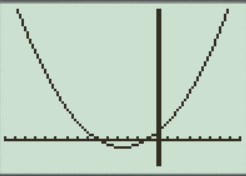This is the perfect viewing window.

d.

When the x -axis ranges from [10,3] and y -axis ranges from [100,20] their graph obtained in the screen is provided below.

Steps to plot the graph of the equation y=x2+7x+6 with the help of graphing utility are as follows:

Step 1: Press MODE key.

Step 2: Use the down arrow key to reach FUNC option.

Step 3: Press ENTER key.

Step 4: Press Y= key.

Step 5: Enter the function y=x2+7x+6 .

Step 6: Press WINDOW key. Change the settings to

Xmin=10Xmax=3Ymin=100Ymax=20

For better view of graph.

Step 8: Press GRAPH key.

The result obtained on the screen is provided below,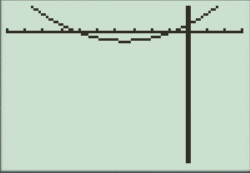This window has large view, in this the graph points are not clear.

So perfect viewing window of the graph y=x2+7x+6 is option ‘c’ [15,8]by[20,100] .

Thus, option ‘c’ [15,8]by[20,100] has a perfect viewing window.

### Have a homework question?

Subscribe to bartleby learn! Ask subject matter experts 30 homework questions each month. Plus, you’ll have access to millions of step-by-step textbook answers!